# Profitability Index

The ratio between the present value of future cash flows to the initial investment

## What is the Profitability Index?

The Profitability Index (PI) measures the ratio between the present value of future cash flows and the initial investment. The index is a useful tool for ranking investment projects and showing the value created per unit of investment.

The Profitability Index is also known as the Profit Investment Ratio (PIR) or the Value Investment Ratio (VIR).### Profitability Index Formula

The formula for the PI is as follows: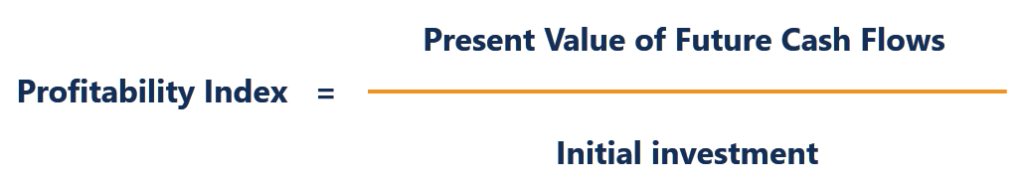or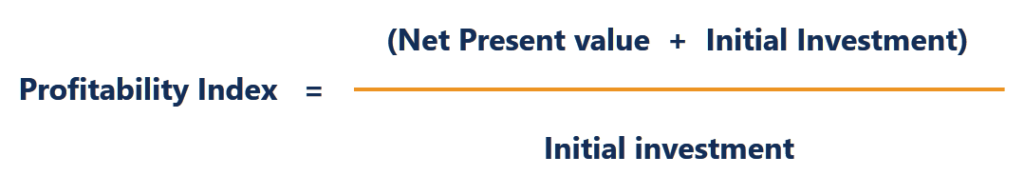Therefore:

• If the PI is greater than 1, the project generates value and the company may want to proceed with the project.
• If the PI is less than 1, the project destroys value and the company should not proceed with the project.
• If the PI is equal to 1, the project breaks even and the company is indifferent between proceeding or not proceeding with the project.

The higher the profitability index, the more attractive the investment.

### Example of Profitability Index

Company A is considering two projects:

Project A requires an initial investment of \$1,500,000 to yield estimated annual cash flows of:

• \$150,000 in Year 1
• \$300,000 in Year 2
• \$500,000 in Year 3
• \$200,000 in Year 4
• \$600,000 in Year 5
• \$500,000 in Year 6
• \$100,000 in Year 7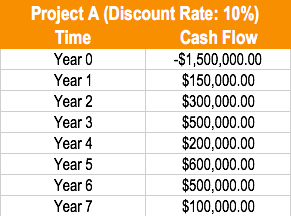The appropriate discount rate for this project is 10%.

Project B requires an initial investment of \$3,000,000 to yield estimated annual cash flows of:

• \$100,000 in Year 1
• \$500,000 in Year 2
• \$1,000,000 in Year 3
• \$1,500,000 in Year 4
• \$200,000 in Year 5
• \$500,000 in Year 6
• \$1,000,000 in Year 7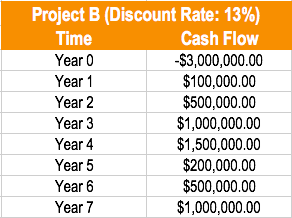The appropriate discount rate for this project is 13%.

Company A is only able to undertake one project. Using the profitability index method, which project should the company undertake?

Using the PI formula, Company A should do Project A. Project A creates value – Every \$1 invested in the project generates \$.0684 in additional value.

#### Discounting the Cash Flows of Project A:

• \$150,000 / (1.10) = \$136,363.64
• \$300,000 / (1.10)^2 = \$247,933.88
• \$500,000 / (1.10)^3 = \$375,657.40
• \$200,000 / (1.10)^4 = \$136,602.69
• \$600,000 / (1.10)^5 = \$372,552.79
• \$500,000 / (1.10)^6 = \$282,236.97
• \$100,000 / (1.10)^7 = \$51,315.81

Present value of future cash flows:

\$136,363.64 + \$247,933.88 + \$375,657.40 + \$136,602.69 + \$372,552.79 + \$282,236.97 + \$51,315.81 = \$1,602,663.18

Profitability index of Project A: \$1,602,663.18 / \$1,500,000 = \$1.0684. Project A creates value.

#### Discounting the Cash Flows of Project B:

• \$100,000 / (1.13) = \$88,495.58
• \$500,000 / (1.13)^2 = \$391,573.34
• \$1,000,000 / (1.13)^3 = \$693,050.16
• \$1,500,000 / (1.13)^4 = \$919,978.09
• \$200,000 / (1.13)^5 = \$108,551.99
• \$500,000 / (1.13)^6 = \$240,159.26
• \$1,000,000 / (1.13)^7 = \$425,060.64

Present value of future cash flows:

\$88,495.58 + \$391,573.34 + \$693,050.16 + \$919,978.09 + \$108,551.99 + \$240,159.26 + \$425,060.64 = \$2,866,869.07

Profitability index of Project B: \$2,866,869.07 / \$3,000,000 = \$0.96. Project B destroys value.

### Advantages of the Profitability Index

• The profitability index indicates whether an investment should create or destroy company value.
• It takes into consideration the time value of money and the risk of future cash flows through the cost of capital.
• It is useful for ranking and choosing between projects when capital is rationed.

Example: A company allocates \$1,000,000 to spend on projects. The initial investment, present value, and profitability index of these projects are as follows: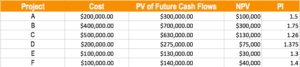The incorrect way to solve this problem would be to choose the highest NPV projects: Projects B, C, and F. This would yield an NPV of \$470,000.

The correct way to solve this problem would be to choose the projects starting from the highest profitability index until cash is depleted: Projects B, A, F, E, and D. This would yield an NPV of \$545,000.

### Disadvantages of the Profitability Index

1. The profitability index requires an estimate of the cost of capital to calculate.
2. In mutually exclusive projects where the initial investments are different, it may not indicate the correct decision.

Thank you for reading this CFI guide. To continue learning, you may find the CFI resources listed below helpful.  CFI’s mission is to help anyone advance their career through the Financial Modeling & Valuation Analyst program.